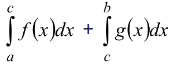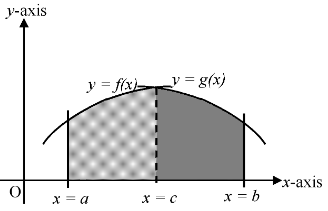# Introduction

## Application of Integrals of Class 12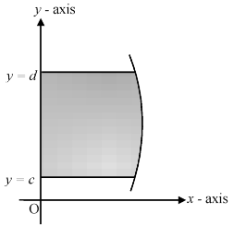(iii) Let f (x) be a continuous monotonic function in [a, b] such that f (x) ≤ 0 in [a, c] and f (x) ≥ 0 in [c, b]. Then the area bounded by the curve y = f (x), x - axis and ordinates x = a and x = b is given by the formula.

 A =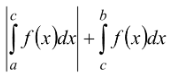or A = −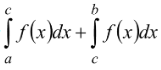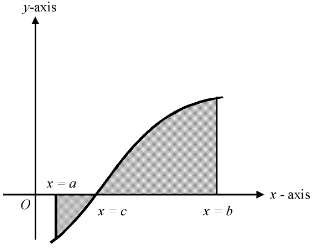(iv) If we have two functions f (x) and g (x) such that f (x) ≤ g (x) in [a, b], then area bounded by the curves y = f (x), y = g (x) and ordinates x = a and x = b is given by A =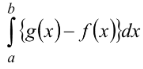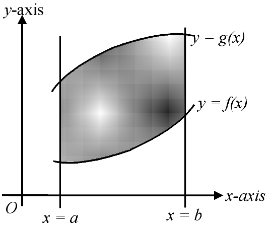(v) A =